# Creating a map with contour lines

Plotting a contour map is handled by `pygmt.Figure.grdcontour`.

```import pygmt

# Load sample earth relief data
grid = pygmt.datasets.load_earth_relief(resolution="05m", region=[-92.5, -82.5, -3, 7])
```

## Create contour plot

The `pygmt.Figure.grdcontour` method takes the grid input. It plots annotated contour lines, which are thicker and have the elevation/depth written on them, and unannotated contour lines. In the example below, the default contour line intervals are 500 meters, with an annotated contour line every 1,000 meters. By default, it plots the map with the equidistant cylindrical projection and with no frame.

```fig = pygmt.Figure()
fig.grdcontour(grid=grid)
fig.show()
```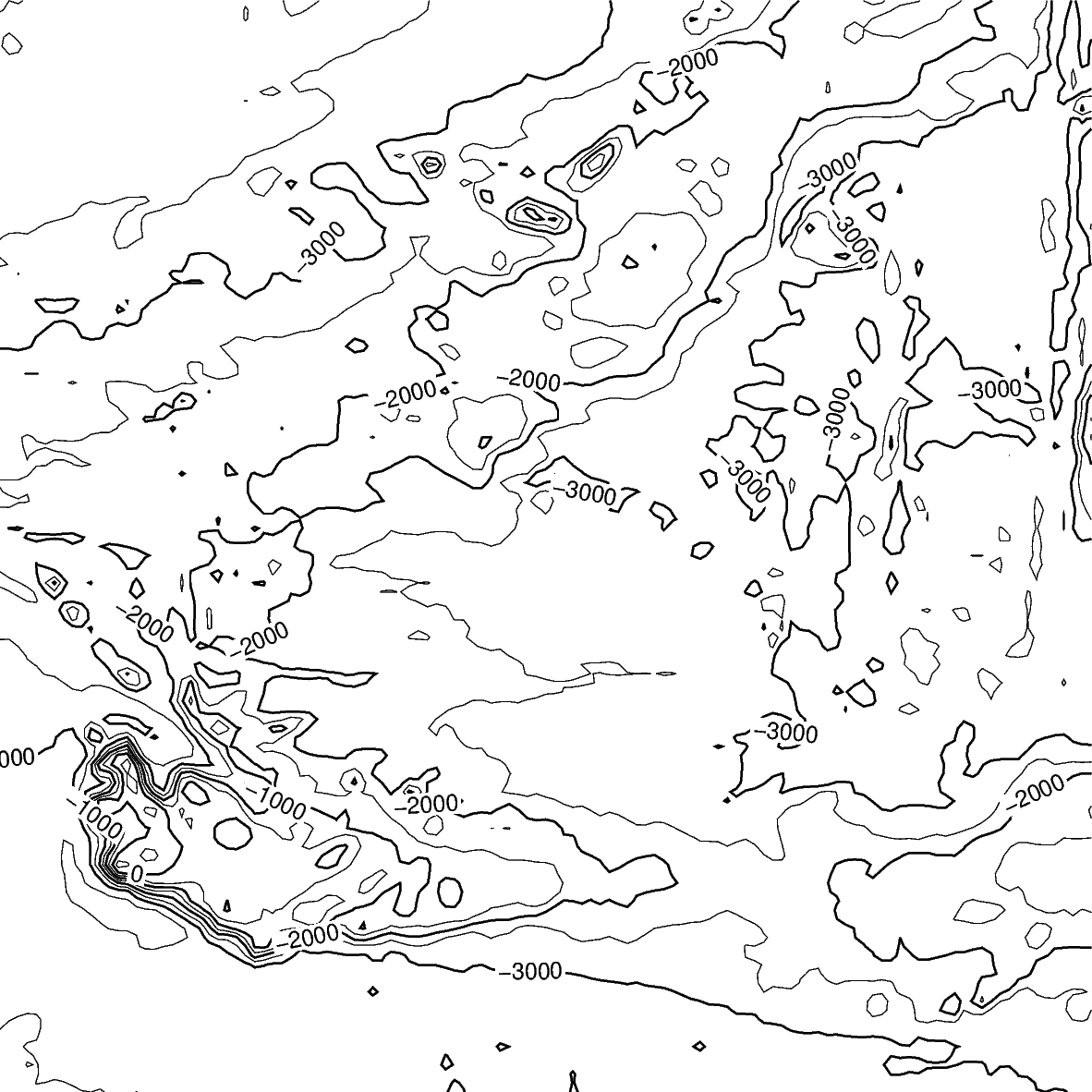## Contour line settings

Use the `annotation` and `interval` parameters to adjust contour line intervals. In the example below, there are contour intervals every 250 meters and annotated contour lines every 1,000 meters.

```fig = pygmt.Figure()
fig.grdcontour(
annotation=1000,
interval=250,
grid=grid,
)
fig.show()
```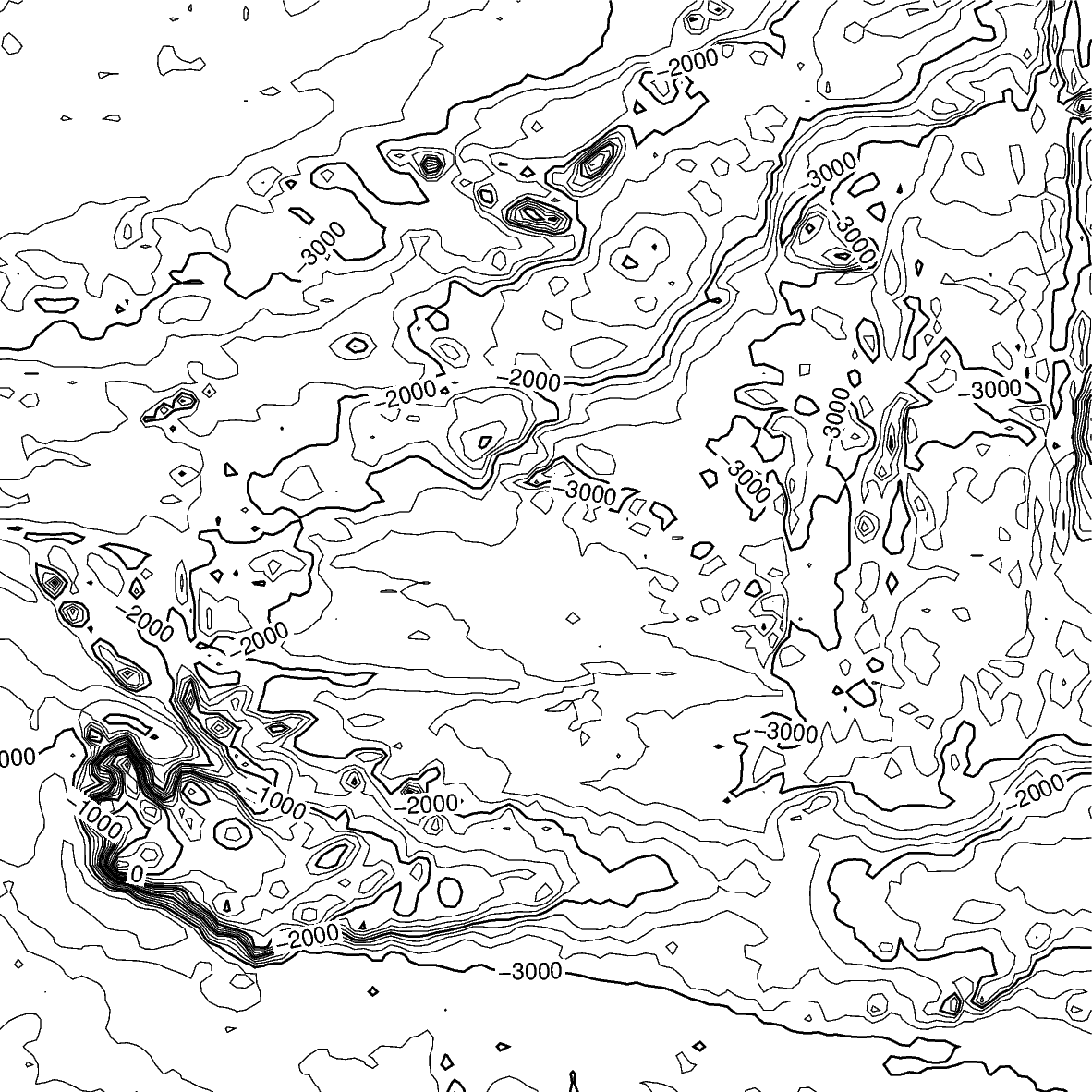## Contour limits

The `limit` parameter sets the minimum and maximum values for the contour lines. The parameter takes the low and high values, and is either a list (as below) or a string `limit="-4000/-2000"`.

```fig = pygmt.Figure()
fig.grdcontour(
annotation=1000,
interval=250,
grid=grid,
limit=[-4000, -2000],
)
fig.show()
```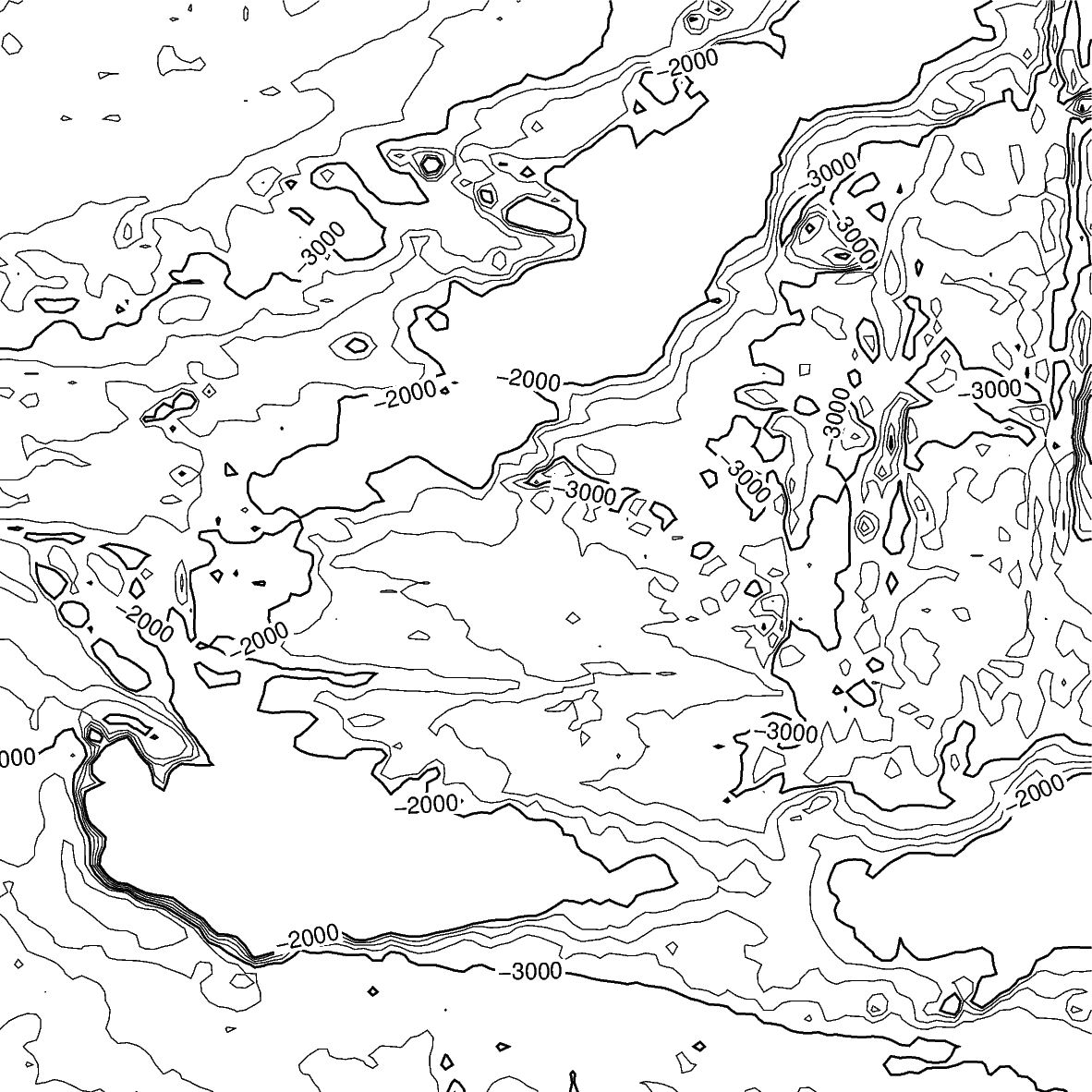## Map settings

The `pygmt.Figure.grdcontour` method accepts additional parameters, including setting the projection and frame.

```fig = pygmt.Figure()
fig.grdcontour(
annotation=1000,
interval=250,
grid=grid,
limit=[-4000, -2000],
projection="M10c",
frame=True,
)
fig.show()
```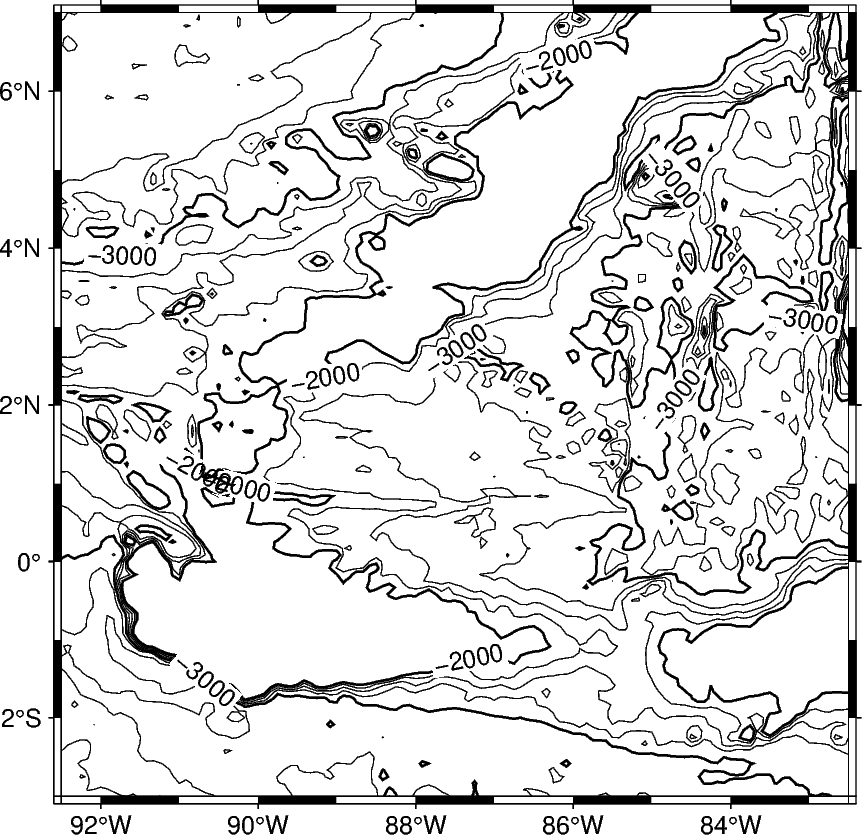The `pygmt.Figure.grdimage` method can be used to add a colormap to the contour map. It must be called prior to `pygmt.Figure.grdcontour` to keep the contour lines visible on the final map. If the `projection` parameter is specified in the `pygmt.Figure.grdimage` method, it does not need to be repeated in the `pygmt.Figure.grdcontour` method. Finally, a colorbar is added using the `pygmt.Figure.colorbar` method.

```fig = pygmt.Figure()
fig.grdimage(
grid=grid,
cmap="haxby",
projection="M10c",
frame=True,
)
fig.grdcontour(
annotation=1000,
interval=250,
grid=grid,
limit=[-4000, -2000],
)
fig.colorbar(frame=["x+lelevation", "y+lm"])
fig.show()
```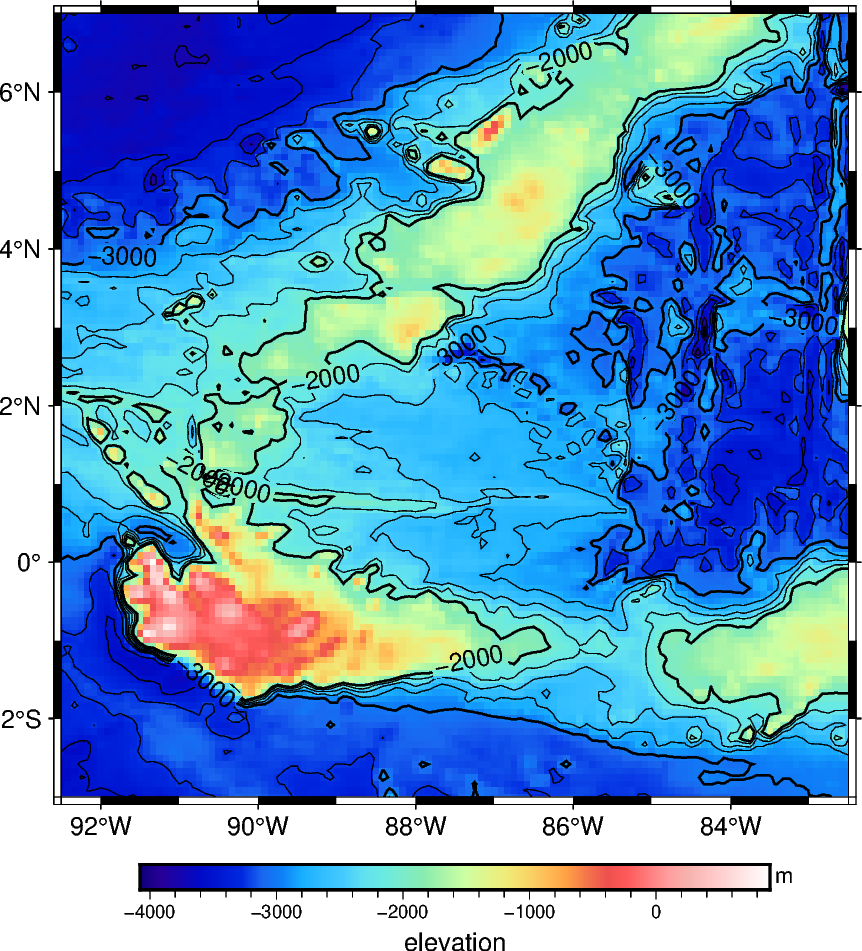Total running time of the script: ( 0 minutes 3.894 seconds)

Gallery generated by Sphinx-Gallery